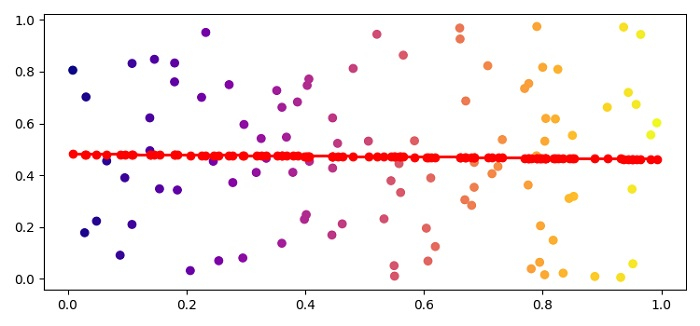# How can I draw a scatter trend line using Matplotlib?

To draw a scatter trend line using matplotlib, we can use polyfit() and poly1d() methods to get the trend line points.

## Steps

• Set the figure size and adjust the padding between and around the subplots.

• Create x and y data points using numpy.

• Create a figure and a set of subplots.

• Plot x and y data points using numpy.

• Find the trend line data points using polyfit() and poly1d() method.

• Plot x and p(x) data points using plot() method.

• To display the figure, use show() method.

## Example

import numpy as np
from matplotlib import pyplot as plt

plt.rcParams["figure.figsize"] = [7.50, 3.50]
plt.rcParams["figure.autolayout"] = True

x = np.random.rand(100)
y = np.random.rand(100)
fig, ax = plt.subplots()
_ = ax.scatter(x, y, c=x, cmap='plasma')
z = np.polyfit(x, y, 1)
p = np.poly1d(z)
plt.plot(x, p(x), "r-o")

plt.show()

## Output